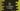# 3 ways to find if a HashSet is empty or not in Dart## Different ways to find if a HashSet is empty or not in Dart:

There are different ways in HashSet to check if it is empty or not in Dart. Dart HashSet provides a couple of properties to check if a HashSet is empty or not.

### Method 1: How to check if a HashSet is empty with the isEmpty property:

The isEmpty property of HashSet is used to check if a HashSet is empty or not. This property is defined as:

``isEmpty → bool``

This is a boolean property. It returns true if the HashSet is empty, else it returns false.

Let’s try this with an example:

``````import 'dart:collection';

void main() {
final firstSet = HashSet.of([1, 2, 3]);
final secondSet = HashSet();

print("firstSet isEmpty: \${firstSet.isEmpty}");
print("secondSet isEmpty: \${secondSet.isEmpty}");
}``````

In this program, we are checking the isEmpty values of firstSet and secondSet. Here, secondSet is the empty set. If you run this program, it will print:

``````firstSet isEmpty: false
secondSet isEmpty: true``````

As you can see, the value of isEmpty is true for the empty HashSet.

### Method 2: By using the isNonEmpty property:

The isNonEmpty property returns one boolean value based on if the HashSet is not empty or not. This property is defined as:

``isNotEmpty → bool``

It returns true if the HashSet is not empty and false otherwise.

Example:

``````import 'dart:collection';

void main() {
final firstSet = HashSet.of([1, 2, 3]);
final secondSet = HashSet();

print("firstSet isNotEmpty: \${firstSet.isNotEmpty}");
print("secondSet isNotEmpty: \${secondSet.isNotEmpty}");
}``````

This is the same example we used before. It will print:

``````firstSet isNotEmpty: true
secondSet isNotEmpty: false``````

### Method 3: By finding the length of the HashSet:

We can use the length property to find the length of the HashSet. This property is defined as:

``length → int``

It is an integer value. If the length is greater than zero, the HashSet is not empty, else it is.

Example:

``````import 'dart:collection';

void main() {
final firstSet = HashSet.of([1, 2, 3]);
final secondSet = HashSet();

print("firstSet length: \${firstSet.length}");
print("secondSet length: \${secondSet.length}");
}``````

It will print:

``````firstSet length: 3
secondSet length: 0``````

Although we can use the length property to check if a HashSet is empty or not, it is not recommended. Because we already have two predefined boolean properties to check that. The length property is used mainly to find the length of a HashSet.